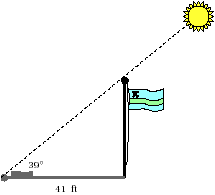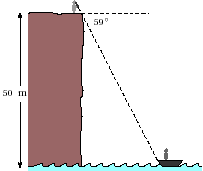/circle/using-slope-angles.html

Adapted from Geometry Labs, by Henri Picciotto

# Using Slope AnglesHow tall is the flagpole? How far is the boat from the base of the cliff?

For the remaining problems, make your own sketches.

1. Looking down at a boat from a 30 meter high lighthouse, an observer measures an angle of 15° below the horizontal. How far is the boat from the base of the lighthouse?

2. A ski-lift rises 200 meters for a run of 250 meters. What angle does it make with the horizontal?

3. At a certain time of day, a 33 ft flagpole casts a 55 ft shadow. What is the angle made by the sun's rays with the horizontal?

4. The banister of a straight staircase makes an angle of 39° with the horizontal. The stairs connect two floors that are 10 feet apart.
1. How much horizontal space does the staircase take?
2. If steps are 8 inches high, how wide are they?

5. You stand on a cliff, looking down at a town in the distance. Using a map, you find that the town is 1.2 kilometers away. The angle your line of vision makes with the horizontal is 11.3°. How high is the cliff?

6. A right triangle has a 15° angle and a short leg of 18 units. How long is the long leg?

7. A right triangle has a 75° angle and a short leg of 18 units. How long is the long leg?

8. The problems in this activity have been rigged to only use angles and slopes that you included in the tables. Explain how to use the 10-cm circle to solve problems of this type involving any angle at all.

9. Find the angles for slopes of 10, 25, 100. What happens as the slope gets bigger and bigger?